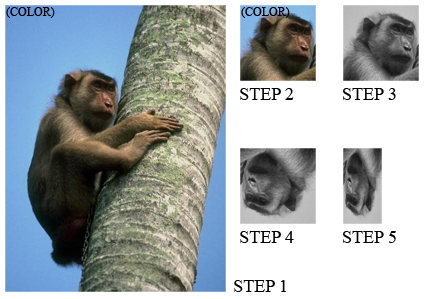# Examples

## Pixel-level Operations

Here are some operations you can do with pixel values, pixel pointers and pixel references:

```rgb8_pixel_t p1(255,0,0);     // make a red RGB pixel
bgr8_pixel_t p2 = p1;         // RGB and BGR are compatible and the channels will be properly mapped.
assert(p1==p2);               // p2 will also be red.
assert(p2!=p1);         // operator[] gives physical channel order (as laid down in memory)
assert(semantic_at_c<0>(p1)==semantic_at_c<0>(p2)); // this is how to compare the two red channels
get_color(p1,green_t()) = get_color(p2,blue_t());  // channels can also be accessed by name

const unsigned char* r;
const unsigned char* g;
const unsigned char* b;
rgb8c_planar_ptr_t ptr(r,g,b); // constructing const planar pointer from const pointers to each plane

rgb8c_planar_ref_t ref=*ptr;   // just like built-in reference, dereferencing a planar pointer returns a planar reference

p2=ref; p2=p1; p2=ptr; p2=rgb8_pixel_t(1,2,3);    // planar/interleaved references and values to RGB/BGR can be freely mixed

//rgb8_planar_ref_t ref2;      // compile error: References have no default constructors
//ref2=*ptr;                   // compile error: Cannot construct non-const reference by dereferencing const pointer
//ptr=p1;                   // compile error: Cannot set the fourth pixel through a const pointer
//p1 = pixel<float, rgb_layout_t>();// compile error: Incompatible channel depth
//p1 = pixel<bits8, rgb_layout_t>();// compile error: Incompatible color space (even though it has the same number of channels)
//p1 = pixel<bits8,rgba_layout_t>();// compile error: Incompatible color space (even though it contains red, green and blue channels)
```

Here is how to use pixels in generic code:

```template <typename GrayPixel, typename RGBPixel>
void gray_to_rgb(const GrayPixel& src, RGBPixel& dst)
{
gil_function_requires<PixelConcept<GrayPixel> >();
gil_function_requires<MutableHomogeneousPixelConcept<RGBPixel> >();

typedef typename color_space_type<GrayPixel>::type gray_cs_t;
static_assert(boost::is_same<gray_cs_t,gray_t>::value, "");

typedef typename color_space_type<RGBPixel>::type  rgb_cs_t;
static_assert(boost::is_same<rgb_cs_t,rgb_t>::value, "");

typedef typename channel_type<GrayPixel>::type gray_channel_t;
typedef typename channel_type<RGBPixel>::type  rgb_channel_t;

gray_channel_t gray = get_color(src,gray_color_t());
static_fill(dst, channel_convert<rgb_channel_t>(gray));
}

// example use patterns:

// converting gray l-value to RGB and storing at (5,5) in a 16-bit BGR interleaved image:
bgr16_view_t b16(...);
gray_to_rgb(gray8_pixel_t(33), b16(5,5));

// storing the first pixel of an 8-bit grayscale image as the 5-th pixel of 32-bit planar RGB image:
rgb32f_planar_view_t rpv32;
gray8_view_t gv8(...);
gray_to_rgb(*gv8.begin(), rpv32);
```

As the example shows, both the source and the destination can be references or values, planar or interleaved, as long as they model `PixelConcept` and `MutablePixelConcept` respectively.

## Resizing image canvas

Resizing an image canvas means adding a buffer of pixels around existing pixels. Size of canvas of an image can never be smaller than the image itself.

Suppose we want to convolve an image with multiple kernels, the largest of which is 2K+1 x 2K+1 pixels. It may be worth creating a margin of K pixels around the image borders. Here is how to do it:

```template <typename SrcView,   // Models ImageViewConcept (the source view)
typename DstImage>  // Models ImageConcept     (the returned image)
void create_with_margin(const SrcView& src, int k, DstImage& result)
{
gil_function_requires<ImageViewConcept<SrcView> >();
gil_function_requires<ImageConcept<DstImage> >();
gil_function_requires<ViewsCompatibleConcept<SrcView, typename DstImage::view_t> >();

result=DstImage(src.width()+2*k, src.height()+2*k);
typename DstImage::view_t centerImg=subimage_view(view(result), k,k,src.width(),src.height());
std::copy(src.begin(), src.end(), centerImg.begin());
}
```

We allocated a larger image, then we used `subimage_view` to create a shallow image of its center area of top left corner at (k,k) and of identical size as `src`, and finally we copied `src` into that center image. If the margin needs initialization, we could have done it with `fill_pixels`. Here is how to simplify this code using the `copy_pixels` algorithm:

```template <typename SrcView, typename DstImage>
void create_with_margin(const SrcView& src, int k, DstImage& result)
{
result.recreate(src.width()+2*k, src.height()+2*k);
copy_pixels(src, subimage_view(view(result), k,k,src.width(),src.height()));
}
```

(Note also that `image::recreate` is more efficient than `operator=`, as the latter will do an unnecessary copy construction). Not only does the above example work for planar and interleaved images of any color space and pixel depth; it is also optimized. GIL overrides `std::copy` - when called on two identical interleaved images with no padding at the end of rows, it simply does a `memmove`. For planar images it does `memmove` for each channel. If one of the images has padding, (as in our case) it will try to do `memmove` for each row. When an image has no padding, it will use its lightweight horizontal iterator (as opposed to the more complex 1D image iterator that has to check for the end of rows). It choses the fastest method, taking into account both static and run-time parameters.

## Histogram

The histogram can be computed by counting the number of pixel values that fall in each bin. The following method takes a grayscale (one-dimensional) image view, since only grayscale pixels are convertible to integers:

```template <typename GrayView, typename R>
void grayimage_histogram(const GrayView& img, R& hist)
{
for (typename GrayView::iterator it=img.begin(); it!=img.end(); ++it)
++hist[*it];
}
```

Using `boost::lambda` and GIL’s `for_each_pixel` algorithm, we can write this more compactly:

```template <typename GrayView, typename R>
void grayimage_histogram(const GrayView& v, R& hist)
{
for_each_pixel(v, ++var(hist)[_1]);
}
```

Where `for_each_pixel` invokes `std::for_each` and `var` and `_1` are `boost::lambda` constructs. To compute the luminosity histogram, we call the above method using the grayscale view of an image:

```template <typename View, typename R>
void luminosity_histogram(const View& v, R& hist)
{
grayimage_histogram(color_converted_view<gray8_pixel_t>(v),hist);
}
```

This is how to invoke it:

```unsigned char hist;
std::fill(hist,hist+256,0);
luminosity_histogram(my_view,hist);
```

If we want to view the histogram of the second channel of the image in the top left 100x100 area, we call:

```grayimage_histogram(nth_channel_view(subimage_view(img,0,0,100,100),1),hist);
```

No pixels are copied and no extra memory is allocated - the code operates directly on the source pixels, which could be in any supported color space and channel depth. They could be either planar or interleaved.

## Using image views

The following code illustrates the power of using image views:

```jpeg_read_image("monkey.jpg", img);
step1=view(img);
step2=subimage_view(step1, 200,300, 150,150);
step3=color_converted_view<rgb8_view_t,gray8_pixel_t>(step2);
step4=rotated180_view(step3);
step5=subsampled_view(step4, 2,1);
jpeg_write_view("monkey_transform.jpg", step5);
```

The intermediate images are shown here:Notice that no pixels are ever copied. All the work is done inside `jpeg_write_view`. If we call our `luminosity_histogram` with `step5` it will do the right thing.